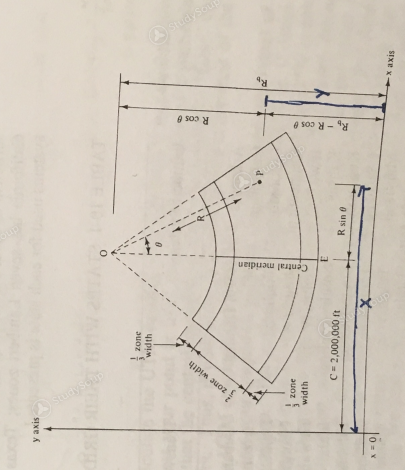×

×

CLEMSON / Civil Engineering / CE 2550 / What does geographic information system means?

# What does geographic information system means? Description

##### Description: These notes cover what will be covered on Geomatics Test 3
0 Pages 56 Views 6 Unlocks
Reviews

CALCULATIONS OF PLANE COORDINATES ON A LAMBERT GRID

We also discuss several other topics like What does abnormal psychology refers to?

In this section, we consider how the geographic coordinates of a point can be transformed into plane coordinates. For a particular Lambert projection zone a central meridian is established near the center of the zone, and is given an x value of 2,000,000 ft.

The x axis is placed well below the southern edge of the zone and is given a y value of zero. Thus all x and y positions in the projection area will be positive. This information is shown in Fig. 10-13 together with the other information needed to transform the geographic coordinates of point P into Lambert grid coordinates.We also discuss several other topics like What is the history of psychobiology?Figure10-13We also discuss several other topics like What is the definition of saccharomyces cerevisiae?

We also discuss several other topics like Who defined mesoamerica?

x = R sin θ + C

y = Rb - R cos θ

Ex: on page 55

Don't forget about the age old question of Name the themes in the study of life.

In the figure point O is the apex of the cone from which the area is projected, line OE is the central meridian and C is the x-coordinate of that meridian, equal to 2,000,000 ft. The meridians are straight lines that converge at point O. The parallels of latitude are arcs of concentric circles that have their centers at point O (see. Figs. 10-8 and 10-13).

Line OP represents part of a meridian through point O and has a length equal to R. The y-coordinate of point O is a constant equal to Rb. The angle θ between lines OE and OP is called the mapping angle or convergence angle.We also discuss several other topics like What makes an adolescent is different in each culture?

The National Geodetic Survey has available for each state using the Lambert projection the necessary information needed to use plane coordinates. Included in this information are values of R for each whole minute of longitude as well as the latitude and longitudes for each of their control stations.

Tables E and F in Appendix A show this type of information. The calculations necessary to compute the state plane coordinates for these stations are illustrated in this section even though the National Geodetic Survey provides these coordinates for many of their stations. From Fig. 10-13 the x- and y- plane coordinates of point P can be seen to equal the following:

x = R sin θ + C

y = Rb - R cos θ

If the angle θ is to the left of the central meridian, it will have a negative value and thus the value of R sin θ will be negative for computing the x coordinate. In computing the y coordinate for point R, cos θ will always be subtracted. Example 10-1 that follows illustrates the determination of the Lambert plane coordinates for a particular station in South Carolina.

South Carolina is divided into two Lambert zones, north and south, both having a central meridian of 81° 00' 00" W. The north zone has standard parallels at latitude 32° 20' and 33° 40' N, as shown in Fig. 10-14, where they are labeled Scale Exact. It should be noted in this figure that the exact division between the north and the south zones is not a line of constant latitude. Rather, the dividing line follows the somewhat irregular county lines. This means that all counties fall completely in one zone or the other.Figure 10-14 State Plane Coordinate Zones for South Carolina

It is considered necessary to make calculations for lane coordinates to at least 10 place accuracy, and this practice is followed in this chapter.

Page ExpiredIt looks like your free minutes have expired! Lucky for you we have all the content you need, just sign up here